# Help for PolyMathLite - Android Version

PolyMathLite is a part of a proven computational system from PolyMath Software that has been specifically created for educational or professional use.  It allows the user to apply effective numerical analysis techniques during interactive problem solving on Android OS smartphones, tablets and computers.

Results are presented with a standard html based POLYMATH Report for easy understanding and can be shared across for incorporation into mail messages, papers, and reports.  Students, engineers, mathematicians, scientists, or anyone with a need to problems will appreciate the efficiency and speed of problem solution.

This Help will enable you to start using PolyMathLite for numerical problem solving. For advanced applications, Please refer also to PolyMathLite User's Manual which is available at the website: www.PolyMathLite.com.  Other more advanced versions of PolyMath are available for personal computers from the website:  www.PolyMathSoftware.comMain Problem Entry Screen

# Main Problem Entry Screen

The main problem entry text box is where the user can enter a new problem or modify an existing one. All problems are entered in plain text format. Four types of problems can be entered; (1) systems of linear equations (2) systems of nonlinear equations (3) systems of first order, ordinary differential equations, and (4) problems involving regression analysis. Each problem type has its own syntax. New users are encouraged to execute some of the sample problems in order to understand problem entry in addition to studying this HELP.  In case the user does not enter the problem correctly, a detailed error message is displayed that guides the user regarding the type of the error and indicates the line number of its occurrence.

To start using PolyMathLite, you first need to determine to what category of problem you want to solve. After that you can select a problem belonging to the same category from the PolyMathLite Sample Problems menu. The sample problem can help you learning the problem entry syntax. By executing the sample problem, you can see the content and the format of the results. The easiest way to enter a new problem is by modifying a similar sample problem. Note that lines starting with the # sign indicate a "comment" which is being ignored by PolyMathLite when solving the problem. After entering a problem, the Solve button is tapped for solving the problem and obtaining the results on the "POLYMATH Report" screen. In case of errors in the problem set up, the "POLYMATH Report" screen will contain only error messages, and some additional information that can be helpful in identifying and correcting the source of the error.

The main entry screen has a standard toolbar under the PolyMathLite icon on the left followed by the "Open", "Sample" and "Run" buttons. To enter a new problem you can start typing on the main entry screen or simply open and modify an existing sample problem. It is recommended that the first line of a problem be started with a comment which contains a short description of the problem. This description is automatically used to identify the problem when saving or retrieving it. You may open options available from your problem display by tapping the three vertical dots icon just above the Ln (Line) indicator on the right side of the PolyMathLite icon on the upper right side of the display.  Tapping on the desired option will select your desired option.  A tap on "Save" will result in saving your problem with the title as the Comment used in Line 1 of your problem.  The disk icon will produce the "Save". Your saved problem will then become available under a depression of the "Open" button..

The syntax of the problem input depends on the category to which the problem belongs. The following explanations deal with the problem input of the various categories.

## PolyMathLite Problem Categories

This section explains the different problem types that can be entered in the PolyMathLite problem entry window and the required syntax for each problem type. The # sign indicates a user comment section which the computer ignores except it is used to name the problem when it is the first line. Any line starting with # anywhere else in the problem is completely ignored by PolyMathLite. Also any line content after # is ignored by PolyMathLite calculations.

Remember that when saving the program, the first line (which must start with the # sign) is used to indicate the problem name.

### (1) System of Only Linear Equations (LEQ)

This category involves the solution of a system of linear algebraic equations. The number of equations must be equal to the number of unknowns. Only the plus, minus, and multiplication operators are allowed. All the variables must appear in linear expressions (no x*x or 1/x). Note that the linear algebraic equations can be entered in any order. (The free version is limited to five linear equations.)

Example 1.1.1

The following example demonstrates the input of a linear system of equations:

 ``` # Example 1.1.1``` ``` # Simple Linear Equations System a + 2*c = 50 b = -2 + c a + 23*c = 12 + b```

The POLYMATH Report includes the values of the unknowns at the solution (a = 54, b = -4 and c = -2, for this problem) and the problem definition in both matrix and system of equations formats.

### (2) System of Nonlinear Equations and Explicit Algebraic Equations (NLE)

The problems belonging to this category contain implicit nonlinear algebraic equations that require iterative solution and explicit algebraic equations where individual variables can be expressed as explicit functions of other variables and constants. An implicit nonlinear equation must be entered in the form:

f(x) = an expression

where x is the chosen variable name, and  f(x)  is an expression that will have the value of zero at the solution.

An explicit equation should be rewritten in the form:

`x = an expression `

where the expression may contain constants, implicit variables, and explicit variables.
Only real roots (non-complex) can be found. Multiple roots are determined for a single equation where the range of the variable must be provided. (The free version is limited to 3 nonlinear and 10 explicit algebraic equations and uses only the NLE - Nwtn2 algorithm.)

### (2.1) System of Only Explicit Algebraic Equations

This type of problem contains a set of explicit algebraic equations. Each equation must start with a variable name followed by the equal sign and a linear or nonlinear algebraic expression. The equations may be entered in any order.  During the solution, the equations are automatically ordered to achieve a solution.  The following system of explicit equations calculates the two roots of a quadratic equation.  Note that both problem setups have the same solution.

Example 2.1.1:

 ``` # Example 2.1.1``` ``` # Explicit System of Nonlinear Equations a = 1 b = -12 c = 35 disc = b^2-4*a*c x1 = (-b+sqrt(disc))/(2*a) x2 = (-b-sqrt(disc))/(2*a) ```

The POLYMATH Report includes the values of the unknowns (x1 = 7, x2 = 5) and all the variables at the solution, a copy of the problem definition in HTML format, and MATLAB formatted version of the problem definition.

HINT! This option for NLEs is very useful for solving systems of explicit algebraic equations for problems of the type that would have been solved using electronic calculators.  Note that the equations do not need to be ordered for solution.  Keep this option in mind for your general explicit calculational needs.

See also: Sample problems 4, 5 advanced sample problems 15 and 16, PolyMathLite Control Buttons, Variables and Expressions

### (2.2) Single Nonlinear Equation and Explicit Algebraic Equations

This option involves the solution of a single implicit nonlinear equation with any number of explicitly defined variables. The implicit nonlinear equation must be arranged to an expression which is equal to zero at the solution. For the variable associated with the implicit equation, both minimal and maximal initial estimates must be provided. Multiple roots are reported if the problem has several solutions (roots) between the minimal and maximal initial estimates. The equations and initial estimates can be entered in any order. PolyMathLite always reorders the equations during the problem solution.

Example 2.2.1:

 ``` # Example 2.2.1``` ``` # Solve the Nonlinear Equation  # x*log(x/2)+4*x^2 = 1620 f(x) = t + 4*x^2 - 1620 t = x*log(x/2) x(min) = 0 y(max) = 100```

The POLYMATH Report includes the value/s of the unknown (x=20, in this case), the function value/s (f(x)= 1.0e-8), the values of all the variables at the solution, a copy of  the problem definition in HTML format, and a MATLAB program m-file for solving the same problem.

See also: Sample problems 6, 7, advanced sample problem 17,  PolyMathLite Control Buttons, and Variables and Expressions.
See also topics in the PolyMathLite Manual (found on the polymathlite.com website): Solution Algorithms and Problem Export to MATLAB.

### (2.3) Nonlinear Equations and Explicit Algebraic Equations

This option involves the solution of a system comprising of several, implicit, nonlinear algebraic equations with any number of explicitly defined variables. All the implicit equations must be rewritten in the form:

f(x) = an expression

where x is a variable that does not appear on the left hand side of any of the explicit equations and the "expression" must be equal to 0 at the solution. Initial estimates must be provided for all the implicit variables using the syntax: x(0) = a value. The equations may be written in any order.

Example:

 ```# Example 2.3 # Solve a System of 2 Nonlinear Equations # 2*x+x*y-y^2 = -290 # x*log(y/2)+x*y = 105 f(x) = 2*x+x*y-y^2 + 290 f(y) = t+x*y - 105 t = x*log(y/2) x(0) = 2 y(0) = 10```

The POLYMATH Report includes the values of the unknowns (x = 5, y = 20), the function values (f(x)= -2.7E-12, f(y)= 1.3E-12 ) and the values of all the additional variable/s at the solution, a copy of the problem definition in HTML format, and a MATLAB program for solving the same problem.

See also: Sample problem 8 advanced sample problems 18, 19, 20 , and 21, PolyMathLite Control Buttons, and Variables and Expressions.
See also (in PolyMathLite manual): Solution Algorithms, and Problem Export to MATLAB

### (2.4) Constrained Nonlinear Equations

A special solution algorithm is available for problems where some of the some of the variables can have only positive values.
For details refer to Sample problem 21 and the PolyMathLite User's Manual.

### Consider a problem containing three linear and two nonlinear equations in the original form:

a+b=c
5a=3+b
a+ln(6c)=3.8907
3b+d=2c+4
0.1e=sin(d*7.5*3.1416/180)

### Rewriting the set of equation for solution as two nonlinear equations that must be equal to zero at the solutions and three explicit expression yields:

 ```# Example 2.5.1 # Simultaneous Linear and Nonlinear Equations f(c)=3.8907-ln(6*c)-a c(0)=2 f(a)=a+b-c a(0) = 2 b=5*a-3 d=2*c+4-3*b e=sin(d*7.5*3.1416/180)/0.1```

### (3) Ordinary Differential Equations (DEQ)

The problems belonging to this category are comprised of systems of simultaneous first-order ordinary differential equations and explicit algebraic equations. The differential equations must be entered in the following form:
`d(x)/d(t) = an expression `

Here t is the independent variable and x is a dependent variable. Note that other independent and dependent variable names are possible. Auxiliary algebraic equations must be entered in the form:

`x = an expression`

where the same naming rules apply.

An expression may contain any combination of constants and variables. However, the auxiliary equations must be explicit (not simultaneous). PolyMathLite will sort and order the equations and will indicate if a simultaneous situation exists (by warning of 'circular dependency').

Initial conditions must be specified for all the variables defined by differential equations, as well as the independent variable. A final value must also be specified for the independent variable. (The free version is limited to 3 first-order ordinary differential equations, 10 explicit algebraic equations and uses only the ODE (RKF45) integration algorithm.)

Example 3.1.1:

 ```# Example 3.1.1 # Simultaneous Ordinary Differential Equations # Simple Set of Consecutive Reactions d(A)/d(t) = -k1*A d(B)/d(t) = k1*A - k2*B d(C)/d(t) = k2*B k1 = 1 k2 = 2 A(0) = 1 B(0) = 0 C(0) = 0 t(0) = 0 t(f) = 3```

The POLYMATH Report for this example includes a Table listing the initial, minimal, maximal, and final values of all the variables over the integration range. In the example shown, the final values are: A = 0.049787, B = 0.047308 and C = 0.902905. A table containing 50 values of the dependent variables between the initial and final values of the independent variable is also displayed. A copy of the problem definition in HTML format and a MATLAB program for solving the same problem are also included in the POLYMATH Report.

The table containing the values of the dependent and independent variables can be exported to plotting utilities to prepare various plots. The use of the Plotim application for this purpose will be demonstrated here. Plotim Free Graphs and Plotim Graph Maker are Android apps available from Google Play.

When tapping the Android menu button (while on the POLYMATH Report screen) enables two standard menu options: Mail and Copy Tables. The results can be plotted by tapping on Copy Tables, opening the Plotim application, and selecting "Paste tab delimited" from the Plotim menu. Then the following plot can be created and displayed: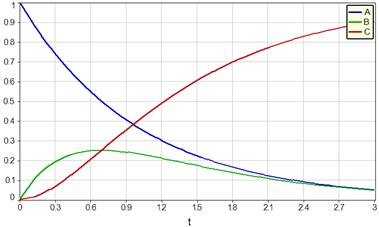Plotim provides various options to manipulate and format the plot.

See also: Sample problems 10, 11, advanced sample problems 22, 23, 24, 25, 26 and 27, PolyMathLite Control Buttons, and Variables and Expressions. See also (in PolyMathLite manual): Plotting Tabular Data, Solution Algorithms, and Problem Export to MATLAB.

### (4.1) Polynomial Regression

PolyMathLite can fit a polynomial of degree n with the general form:

y = P(x) = a0 + a1*x + a2*x^2 + . . . + an*x^n

or a linear equation when the equivalent first degree polynomial is specified

y = P(x) = a0 + a1*x

where a0, a1, ..., an are regression parameters to a set of N tabulated values of x (a single independent variable) versus y (a single dependent variable). The highest degree allowed for a polynomial is N - 1 (thus n >= N - 1). The program calculates the coefficients a0, a1, ..., an by minimizing the sum of squares of the deviations between the calculated y or P(x) and the data for y.

A polynomial regression is specified by the "polyfit" command that must be followed by the name of the independent variable vector, name of the dependent variable vector, a number representing the degree of the polynomial, and an optional string 'origin' which indicates that the polynomial regression curve must go through the origin. These names must be separated by commas.

Example 4.1.1: Correlation of BOD data:

Consider the following BOD (biological oxygen demand) data versus Time:

 Time (days): 1 2 4 6 8 10 12 14 16 18 20 BOD (mg/liter) 0.6 0.7 1.5 1.9 2.1 2.6 2.9 3.7 3.5 3.7 3.8
1. Fit a straight line (linear regression) to the BOD vs. Time data

2. Fit the data to a 2nd degree polynomial

3. Fit the data to a 2nd degree polynomial that passes through the origin Plot the BOD data and the BOD calculated values vs. time and plot the residuals vs. time.

4. Compare the models using R2, variance and 95% confidence interval values

Part 1 Straight Line (Linear Regression)

 # Example 4.1.1 # Linear Regression (Straight Line Fit) # BOD = a0 + a1*Time Time =[1, 2, 4, 6, 8, 10, 12, 14, 16, 18, 20] BOD=[0.6, 0.7, 1.5, 1.9, 2.1, 2.6, 2.9, 3.7, 3.5, 3.7, 3.8] polyfit Time, BOD, 1

This program yields the results a0 = 0.657797 (±0.374896), (95% confidence intervals shown between parentheses), a1 = 0.178056 (±0.031674), R2 = 0.9472735 and the variance: σ2 = 0.0825304.

In the POLYMATH Report a table containing  the observed (measured) y values, the predicted y values (ycalc) and the residuals (r = y- ycalc) are also displayed.

Part 2 Solution

To fit a 2nd degree polynomial, the polyfit statement is revised:

``` polyfit Time, BOD, 2```

The results obtained in this case are a0 = 0.224387 (±0.353054 ), a1 = 0.310403 (±0.081692 ), a2 = -0.006439 (±0.003851), R2 = 0.9815572 and σ2 = 0.0324762.

Part 3 Solution

To fit a 2nd degree polynomial which passes through the origin, the following polyfit statement is used:

``` polyfit Time, BOD, 2, origin```

For this polynomial a0 = 0, by definition, and the rest of the results are: a1 = 0.354779 (±0.044171), a2 = -0.008236 (±0.002724), R2 = 0.9766053 and σ2 = 0.0366186.``` To prepare the required plots, ``` tap the Android menu button (while on the POLYMATH Report screen) and consequently tap the Copy Tables option. Open the Plotim application and select "Paste tab delimited" from its Android menu.``` A plot containing three variables: BOD (the data) BOD calc (calculated values) and Delta BOD (residuals) vs. time should be displayed. To remove the residual plot, tap Edit in Plotim's Android menu and deselect Delta BOD in the variable list. The following plot should appear.```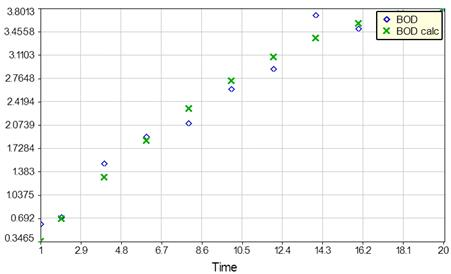To plot the residuals versus Time, tap Edit in Plotim's Android menu and select Delta BOD and deselect BOD and BOD calc in the variable list. The plot is revised: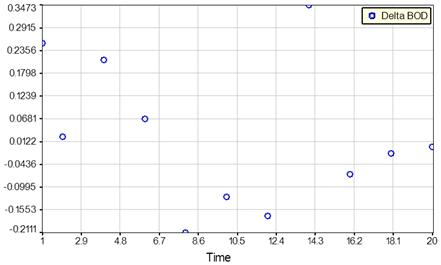Part 4 Solution
Judging by the R2 and σ2 values, the 2nd degree polynomial represents the data the best. However, the confidence interval value of a0 shows that this parameter is not significantly different from zero, thus the use of 2nd degree polynomial passing through the origin should be preferred.

Example 4.1.2: Consider the following set of Pressure (mm Hg) versus Temperature (deg C) data:

 Temperature, T Pressure, P (Deg. C) (mm Hg) -36.7 1 -19.6 5 -11.5 10 -2.6 20 7.6 40 15.4 60 26.1 100 42.2 200 60.6 400 80.1 760

The following operations need to be carried out with these data:

1. Convert the temperature to K (by adding 273.15 to the value shown)

2. Convert the pressure to kPa (by multiplying the value shown by 0.1333)

3. Fit a fourth degree polynomial to the Pressure (dependent variable) vs. Temperature data.

The program to accomplish these tasks is shown below.

 ```# Example 4.1.2 # Transformation of Data # 4th Degree Polynomial Regression # Vapor Pressure Correlation T_C = [-36.7, -19.6, -11.5, -2.6, 7.6, 15.4, 26.1, 42.2, 60.6, 80.1] P_mmHg = [1, 5, 10, 20, 40, 60, 100, 200, 400, 760] T_K = T_C+273.15 P_kPa = P_mmHg*0.1333 polyfit T_K, P_kPa, 4```

The POLYMATH Report includes a Table listing the parameter values and confidence intervals on these values.
For this particular problem the following results are shown.

 Variable Value 95% confidence a0 2121.85 434.312 a1 -33.1504 6.01561 a2 0.196174 0.031053 a3 -0.000522 7.08E-05 a4 5.28E-07 6.02E-08

The POLYMATH Report includes also the statistical metrics: R2, R2adj, root mean squared deviation and variance. A table displaying the observed (measured) y values, the predicted y values (ycalc) and the residuals (r = y- ycalc).

To fit a polynomial that passes through the origin, the "polyfit" command must also include the parameter "origin".
For example:
```     polyfit T_K, P_kPa, 4, origin```

See also: Sample problems 12(a), 12(b) , 12(c), 13, advanced Sample problem 28, PolyMathLite Control Buttons, and Variables and Expressions. See also (in PolyMathLite manual): Plotting Tabular Data.

### (4.2) Multiple Linear Regression

This PolyMathLite option will fit a linear function of the form:

y(x1, x2, ..., xn) = a0 + a1*x1 + a2*x2 + ... + an*xn

where a0, a1, ..., an are regression parameters, to a set of N tabulated values of x1, x2, ..., xn (independent variables) versus y (dependent variable). Note that the number of data points must be greater than or equal to n+1 (thus N >= n+1). The program calculates the coefficients a0, a1, ..., an by minimizing the sum of squares of the deviations between the calculated and the data for y.

Use the mlinfit command to request a multiple linear regression fit. The arguments are the independent variables vector's names, followed by the independent vector name. Additional optional (last) argument is the string 'origin' which indicates that the regression curve should pass through the origin (thus a0 = 0).

Example 4.2.1: Simple Multiple Linear Regression:

The following data are for the wear of a bearing y that may be dependent upon x1 = oil viscosity and x2 = load data.:

y :  293  230   172   91   125   125

x1:  1.61  15.5  22   45   33  40

x2:  851  820  1058  1201  1357  1115

Compare the linear regression models that includes x1 and x2 as independent variables with a model that includes only x1.

 ``` # Example 4.2.1 # Simple Multiple Linear Regression y=[293, 230, 172, 91, 125, 125] x1=[1.61, 15.5, 22, 45, 33, 40] x2=[851, 820, 1058, 1201, 1357, 1115] mlinfit x1, x2, y```

The results obtained in this case are a0 = 360.836 (±118.076), a1 = -3.75246(±1.774), a2 = -0.084265(± 0.140313), R2 = 0.9835209 and σ2 = 159.1951.

To remove x2 from the regression model, the mlinfit statement is revised:

``` mlinfit x1, y```

After this modification the following results are obtained: a0 = 292.784(±37.3385 ), a1 = -4.58727(±1.23994), R2 = 0.963462 and σ2 = 264.7302 .
Judging by the
``` ``` R2``` and ``` σ2``` ``` values, the regression model that contains both x1 and x2 represents the data better. However, the confidence interval value of a2 shows that this parameter is not significantly different from zero, thus removal of x2 from the model should be preferred`.`

Example 4.2.2: Consider the "vapor pressure" example. This time we want to fit to these data a regression model of the form:
log(P_kPa) = a0+a1/T_K +a2*log(T_K)

The dependent variable can be defined by logP=log(P_kPa) and the independent variables defined by OneonT=1/T_K and logT=log(T_K).  Then  mlinfit can be used to find the parameter values.

 ```# Example 4.2.2 # Data Transformations # Multiple Linear Regression T_C = [-36.7, -19.6, -11.5, -2.6, 7.6, 15.4, 26.1, 42.2, 60.6, 80.1] P_mmHg = [1, 5, 10, 20, 40, 60, 100, 200, 400, 760] T_K = T_C+273.15 P_kPa = P_mmHg*0.1333 logP = log(P_kPa) OneonT = 1/T_K logT = log(T_K) mlinfit OneonT, logT, logP```

The POLYMATH Report includes a Table listing the parameter values and confidence intervals on these values. For this particular problem the following results are shown.

 Variable Value 95% confidence a0 77.2682 14.573 a1 -5027.61 629.568 a2 -23.9603 5.03175

See also: Sample Problem 14, advanced Sample Problems 31(a) and 31(b), PolyMathLite Control Buttons and Variables and Expressions.

### (4.3) Nonlinear Regression

Nonlinear regression involves a general mathematical function (model) of the form:

y = f (x1, x2, ..., xn, a0, a1, a2, ..., am)

where a0, a1, ..., an are regression parameters to a set of N tabulated values of x1, x2, ..., xn (independent variables) versus y (dependent variable). Note that the number of data points must be greater than m + 1 (thus N >= m + 1).

Use the nlinfit command to request nonlinear regression fit. The argument is the nonlinear regression model: the dependent variable vector name on the left side of the "=" sign, the independent variable vectors names and the parameters on the right side. Initial estimates must be provided for all the parameter values using expressions of the form:

m(ai) = value

Example 4.3.1: Consider the data for the "vapor pressure example". A nonlinear (Antoine) equation of the form: log(P) = A-B/(T+C).
where P is pressure (mmHg) , T is temperature (deg C) and A, B and C are parameters, need to be fitted to the "vapor pressure" example's data.
Following is the program for finding the optimal parameter values with nlinfit.

 ```# Example 4.3.1 # Data Transformation # Nonlinear Regression # Antoine Equation Utilized logP = A-B/(C+T) T = [-36.7, -19.6, -11.5, -2.6, 7.6, 15.4, 26.1, 42.2, 60.6, 80.1] P = [1, 5, 10, 20, 40, 60, 100, 200, 400, 760] logP = ln(P) nlinfit logP = A-B/(C+T) # initial guess for A, B, C m(A) = 8 m(B) = 2000 m(C) = 200```

The optimal solution obtained is A = 5.76735, B = 677.094 and C = 153.885.

## The Main Problem Entry Screen

The main problem entry screen has the following 3 standard buttons:

 Open Opens a list of previously saved problems. Sample Opens the built in sample problems screen. Run Solves the current problem and presents the POLYMATH Report.

The main toolbar also indicates the current line number Ln #. This is useful to track lines with errors identified after the "Run" button is tapped.

The following menu items are available:

 New Creates a new blank problem Help Opens the Help documentation screen Save Save the current problem on the internal disk of the device. Note that the first line of the problem to be saved must start with the # sign followed by the name of the problem.. The saved problem is given a unique ID number. This ID along with the date at which the problem was last saved appears in the information bar below the extended buttons strip. Share Shares the current problem text with an external Android application such as email, text viewers, cloud storage devices etc. Settings Opens the settings screen which allows changing the parameters of the numerical solution algorithms, such as error tolerances, number of iterations allowed, number of digits shown in the result tables, solution techniques etc. Delete Deletes the current file (if it is stored on the device), and clears the current problem screen to enable new problem entry. To clear the problem entry screen without deleting the displayed file use the Android menu option (clicking the physical menu button of the device ). Increase Font Increases the default font size for the problem entry text editor Decrease Font Decreases the default font size for the problem entry text editor

If the problem definition contains a vector (in regression problems) two additional menu items: "Copy Vectors" and "Paste Vectors" become available. These options enable copying vectors into MS Office like spreadsheet editors, such as Excel, to easily edit long vectors, and then, copy them back into PolyMathLite.

## The Open Saved Problems Screen

The following menu items are displayed on the open screen:

 Order by Name Sorts the displayed problems by name. Order by Date Sorts the displayed problems by the date they were saved. Export Programs DB Exports the entire problems database into a backup file. Import Programs DB Imports the entire problems database from a backup file to become the main problems DB. This option overwrites any existing problems which might be saved currently. Go Back Returns to the last screen.

Additional notes when exporting/importing the entire database problems:

1. Be extremely careful when importing the DB file as it'll overwrite you entire problems database.
2. The backup DB file, where exporting the problems to, or importing the DB from, is located in {Internal Storage}\PolyMathLite\Data_Backup.db on the device internal storage. This file remains there even if you uninstall the application.
3. When exporting the problems library to the backup file above, if a backup file already exists, it will be first copied to a time stamped backup file.
4. When importing the problems library from a backup file, the current database file will first be copied to a time stamped backup file.
5. Import will always take the file named: \PolymathLite\Data_Backup.db and will ignore any of the time-stamped backup files. The time-stamped backup files could be renamed to \PolymathLite\Data_Backup.db for importing them.

## The POLYMATH Report Screen

The following menu items are displayed on the POLYMATH Report screen:

 Share... Enables sharing the POLYMATH Report with external Android applications such as email, text viewers, cloud storage devices etc. Copy Tables The "Copy Tables" option can be used to copy tables displayed within the report. These can be pasted into external application such as Excel to review the tabular data and/or construct charts. Go Back Returns to the last screen.

# Built in Samples Screen

Viewing and running the built in PolyMathLite sample problems is the best way to become familiar with the problem input and execution of PolyMathLite problems. The following problem types are displayed.

 Icon Symbol Type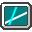LEQ Linear Equations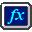NLE Explicit Equations SetNLE Single Nonlinear EquationNLE Multiple Nonlinear EquationsNLE Constrained Multiple Nonlinear Equations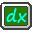DEQ Ordinary Differential Equations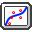REG Polynomial RegressionREG Multiple Linear RegressionREG Nonlinear Regression

# Variables and Expressions

The following objects compose a valid PolyMathLite math expression:

 Expression Objects Description Number A number can be represented in decimal notation or engineering notation. Engineering notation can be used to indicate powers of 10 using 'e' or 'E' . For example, 0.123e-5 is the same as 0.123*10^(-5). Note that only the period "." is used as the decimal delimiter symbol and comma nor spaces must not be used to indicate digits grouping.Sample valid PolyMathLite numbers: 1.0, 2300, 5E-7, 1.23E+9, 34.0046 Invalid PolyMathLite numbers: 5,000.00 12,9 12 000 000 Variable Name Variable names must begin with an alphabetical character and can contain alphabetic and numeric characters or underscore "_". Variable names are case-sensitive, i.e. 't' and 'T' are two different variables. Special characters such as \$, &, #, @ etc. are not allowed.Samples: x1, x2, A12, T, tm4eee, VH2O, PmmHg, KL_1 Arithmetic Operator Returns the arithmetic operations of the binary components. For example '2+3' returns 5 . The '^' symbol is used for exponentiation. The +, -, *, / symbols are used for addition, subtraction, multiplication, and division respectively. The precedence of operators is ^, (* or /), (+ or -) which is invoked working from left to right in an expression.Samples: +, -, *, /, ^ (1 + 2 * 3 ^ 2 ^ 3 / 2 - 4) is equivalent to (1 + (2 * (((3 ^ 2) ^ 3) / 2)) - 4) Function Name Various mathematical function names are available. Examples: sin, cos, exp, ... The full list of available PolyMathLite functions is presented below.Samples: 12+sin(4) exp( 5*9+log(8)) Condition Operator These operators return "1" if the condition met, and "0" if the condition does not meet. Examples: The expression '3>6' returns 0. The expression '4>=4' returns 1.Valid conditional operators: >, <, >=, <=, == Boolean Operator The Boolean operators return "1" if the condition is met, and "0" if the condition is not met. Example: The expression '(2>3) or (1>0)' returns 1. The expression '1 and 0' returns 0.Valid Boolean operators: And, Or If-Then-Else There are three case-insensitive keywords which compose the if-then-else expression structure.  The three keywords are: If, Then, Else. Samples: If (2>5) Then (sin(12)+4) Else (sin(12)+8) if(2>5)then(sin(12)+4)else(sin(12)+8) Parenthesis Open Parenthesis: ( Close Parenthesis: )Sample: x-(2+3)

A special 'if' statement is available, with the following syntax:

 ```if (condition) then (expression1) else (expression2) ```

The parentheses are optional.
The condition may include the following operators: and, or (Boolean operators), > (greater than), < (less than), >= (greater than or equal), <= (less than or equal), == (equals).
The expressions may be any formula, including another 'if' statement.

For example:

 ```A = if (x>0) then (log(x)) else(0) b = if (TmaxT) then (maxT) else (T)) Vol_h1 = if (a>5 and c<2) then 1.12 else 7.89 ```

# Available Functions

A number of standard functions are available for use in the various programs. The arguments of the functions must be enclosed in parentheses. The arguments may be themselves expressions or other functions. The nesting of functions is allowed.

All function names must be given in lower-case letters. The trigonometric functions require that their arguments be given in radians. Conversely, the inverse trigonometric functions give their results in radians.

You should note that the functions require that their arguments be enclosed in parentheses, but that the arguments do not have to be simple numbers. You may have a complicated expression as the argument for a function, and you may even nest the functions, using one function (or an expression including one or more functions) as the argument for another.

# PolyMathLite Capabilities

 Module Limitation Free Version Paid Version LEQ Max # of simultaneous linear equations 4 No limit NLE Max # of simultaneous nonlinear equations 3 No limit NLE Max # of simultaneous explicit equations 10 No limit NLE Number of available solution algorithms 1 4 DEQ Max # of simultaneous differential equations 3 No limit DEQ Max # of simultaneous explicit equations 10 No limit DEQ Number of available solution algorithms 1 (RKF45) 5 stiff and nonstiff REG Max # of data points 24 No limit REG Max polynomial regression degree 5 12 REG Number of nonlinear regression solution algorithms None 2